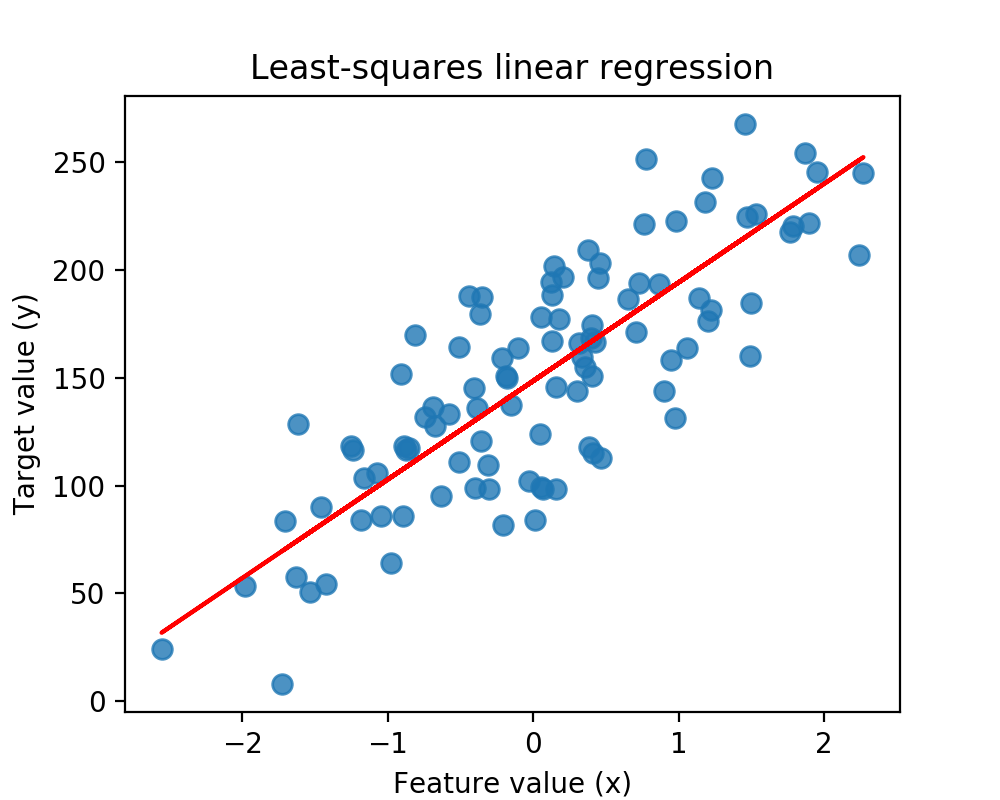# Least Squares Linear Regression

Linear models are one of the simplest kinds of Supervised Learning models. A linear model expresses the target output value in terms of a sum of weighted input variables.

For example, the expected market value of a house might be expressed as:

$$\hat{y_{price}}=212,000 + 109 X_{tax} - 2000 X_{age}$$

Then, a house with feature values $(x_{tax}, x_{age})$ = $(10000, 75)$ would have a predicted selling price of:

$$\hat{y_{price}}=212,000 + 109 \times 10,000 - 2000 \times 75 = 1,152,000$$

### Linear Regression Model

More precisely, a linear model consists of the following parameters.

Feature Vector

$$x = (x_0, x_1, …, x_n)$$

Predicted Output

$$\hat{y} = \hat{w_0} x_0 + \hat{w_1} x_1 + … + \hat{w_n} x_n + \hat{b}$$

Parameters to estimate

Feature weights/model coefficients: $$\hat{w} = (\hat{w_0}, …, \hat{w_n})$$

Constant bias term/intercept: $$\hat{b}$$

### A Linear Regression Model with one Variable (Feature)

Input Instance

$$\textbf{x} = (x_0)$$

Predicted Output

$$\hat{y}=\hat{w_0}x_0 + \hat{b}$$

Parameters to Estimate

Slope:

$$\hat{w_0}$$

Y-intercept (or “bias”):

$$\hat{b}$$

### How are the linear regression parameters $w$, and $b$ estimated?

• Parameters are estimated from training data.
• There are many different ways to estimate $w$ and $b$:
• Differen methods correspond to different “fit” criteria and goals and means of controlling model complexity.
• The learning algorithm finds the parameters that optimize an objective function, typically to minimize some kind of lose function of the predicted target values versus actual target values.

### Least-Squares Linear Regression (“Ordinary least-squares”)

• Finds $w$ and $b$ that minimizes the sum of squared differences (RSS) over the training data between predicted target and actual target values, AKA, mean squared error of the linear model.
• No parameters to control model complexity.
• No matter the values of $w$ and $b$, the result will always be a straight line.

$$RSS(w,b)=\sum_{(i=1)}^N (y_i-(w \cdot x_i + b))^2$$%matplotlib notebook

import numpy as np
import matplotlib
import pandas as pd
import sklearn

print('       Numpy version ' + str(np.__version__))
print('  Matplotlib version ' + str(matplotlib.__version__))
print('      Pandas version ' + str(pd.__version__))
print('     Sklearn version ' + str(sklearn.__version__))

       Numpy version 1.18.1
Matplotlib version 3.1.2
Pandas version 1.0.0
Sklearn version 0.22.1

import matplotlib.pyplot as plt

from sklearn.linear_model import LinearRegression

X_R1, y_R1 = make_regression(n_samples = 100, n_features=1,
n_informative=1, bias = 150.0,
noise = 30, random_state=0)

X_train, X_test, y_train, y_test = train_test_split(X_R1,
y_R1,
random_state=0)
linreg = LinearRegression().fit(X_train, y_train)

print('linear model coeff (w): {}'
.format(linreg.coef_))
print('linear model intercept (b): {:.3f}'
.format(linreg.intercept_))
print('R-squared score (training): {:.3f}'
.format(linreg.score(X_train, y_train)))
print('R-squared score (test): {:.3f}'
.format(linreg.score(X_test, y_test)))

linear model coeff (w): [45.70870465]
linear model intercept (b): 148.446
R-squared score (training): 0.679
R-squared score (test): 0.492


The R-squared values above scores the quality of the regression model, in the same way as the K-nearest neighbors post.

plt.figure(figsize=(5,4))
plt.scatter(X_R1, y_R1, marker= 'o', s=50, alpha=0.8)
plt.plot(X_R1, linreg.coef_ * X_R1 + linreg.intercept_, 'r-')
plt.title('Least-squares linear regression')
plt.xlabel('Feature value (x)')
plt.ylabel('Target value (y)')
plt.show()

<IPython.core.display.Javascript object>Linear models make strong assumptions about the structure of the data. Specifically, linear models assume that the target value can be predicted using a weighted sum of the input variables.